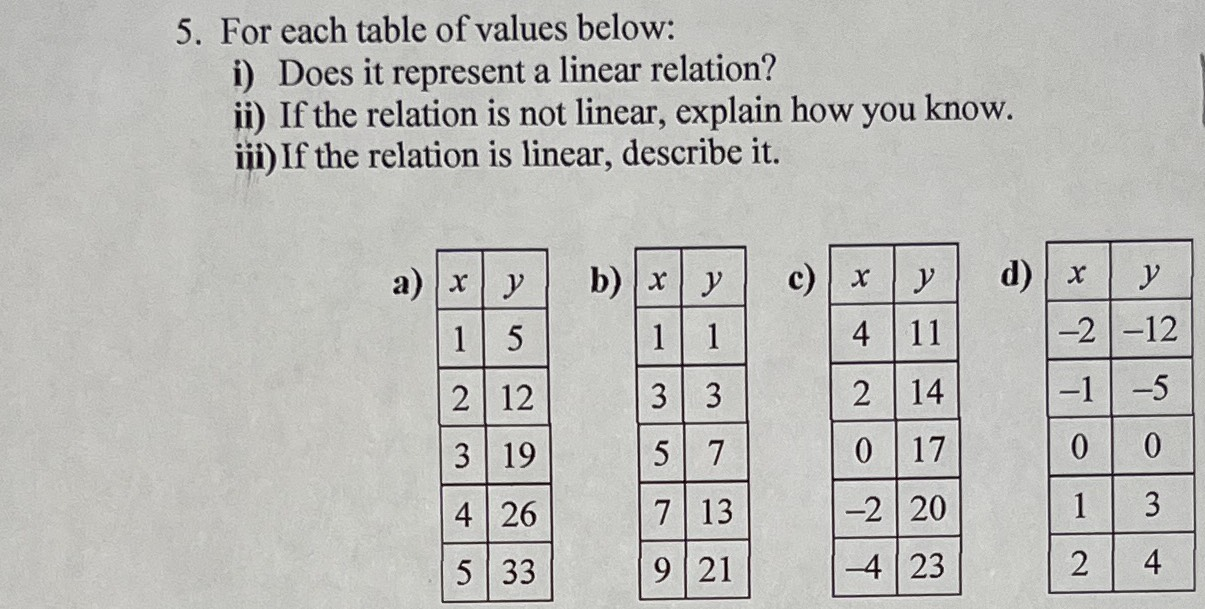### Still have math questions?

Algebra
QuestionFor each table of values below: i) Does it represent a linear relation? ii) If the relation is not linear, explain how you know. iii) If the relation is linear, describe it.

b)No,It doesn't represent l linear relation. $$\frac{3- 1}{3- 1}\ne\frac{7- 3}{5- 3}$$
c)No,It doesn't represent l linear relation. $$\frac{3- 0}{1- 0}\ne \frac{4- 3}{2- 1}$$